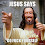## Thursday, October 9, 2014

### NFL Picks Contest- Week 5 Results and Standings

Here are the Week 5 results and standings in the NFL Picks Contest. OneAndDone and ggrouchie put a little daylight between themselves and the pack.

angerisagift- Pittsburgh (W 17-9) = 18 pts.
MOJO- New Orleans (W 37-31) = 16 pts.
Vegas Vic- New Orleans (W 37-31) = 16 pts.
Captain Crunch- Philadelphia (W 34-28) = 16 pts.
OneAndDone- San Diego (W 31-0) = 41 pts.
mrben09- New Orleans (W 37-31) = 16 pts.

ggrouchie- San Diego (W 31-0) = 41 pts.
Coach- Seattle (W 27-17) = 20 pts.
TeamMiRketti- Philadelphia (W 34-28) = 16 pts.
lightning36- New Orleans (W 37-31) = 16 pts.
Neophyte- San Diego (W 31-0) = 41 pts.
Music City Momma- New Orleans (W 37-31) = 16 pts.

TwoMinuteWarning- Philadelphia (W 34-28) = 16 pts.
Grange95-  New Orleans (W 37-31) = 16 pts.
Fullhouse538-
M Prosk- San Diego (W 31-0) = 41 pts.
cokeboy99- New Orleans (W 37-31) = 16 pts.

Here are the current standings;

OneAndDone- 27+17+17+34+41 = 136 pts.
ggrouchie- 27+17+17+34+41 = 136 pts.
Coach- 27+17+21+29+20 = 114 pts.
Vegas Vic- 27+17+21+29+16 = 110 pts.
MOJO-  27+17+17+29+16 = 106 pts.
Captain Crunch- 27+17+17+29+16 = 106 pts.

lightning36- 27+17+17+29+16 = 106 pts.
mrben09- 27+17+17+29+16 = 106 pts.
Music City Momma- 13+17+21+29+16 = 96 pts.
TwoMinuteWarning- (3)+17+37+29+16 = 96 pts.
cokeboy99- 27-(8)+21+34+16 = 90 pts.
Grange95- (3)+17+21+29+16 = 80 pts.

TeamMiRketti- 27+17+17-(3)+16 = 74 pts.
M Prosk- (3)+ 0+ 0+34+41 = 72 pts.
Neophyte- 27+17-(19)+0+41 = 66 pts.
Fullhouse538- (13)+17+17-(3)+0 = 18 pts.

Thanks for playing.

Week 6 picks are due by Saturday at 6pm (CDT). Only Sunday and Monday games are eligible.

Good luck!

#### 1 comment:

1.Ravens thx. hopefully u get my gift sooooooooon. didnt think it would take this long.i thought the pony express was in the past